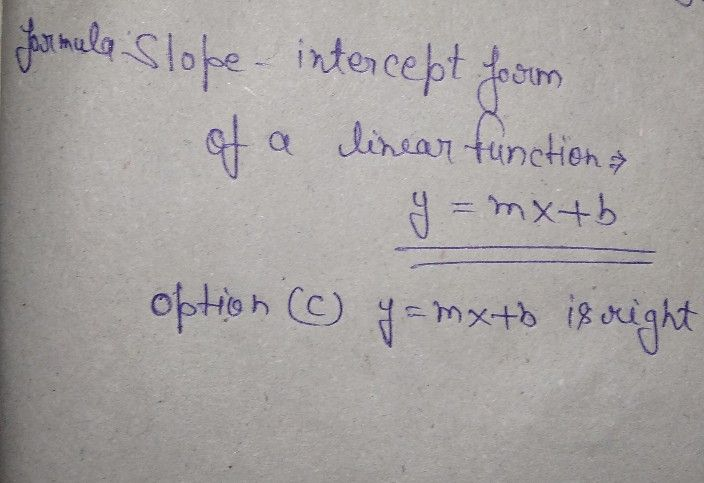Symbol
Problemb. How much would it cost to rent $24$ square feet of space? Post Test Choose the letter of the correct answer. Write the chosen letter on a separate sheet of paper. $1.$ What is the $slope-intercept$ form of a Linear Function? A. $ax+by=c$ C. $y=mx+b$ B. $ax+by+c=0$ D. $y-y_{1}=m\left(x-x_{1}\right)$
7th-9th grade
Algebra
Search count: 107
Question content
Please Answer this is my last coin. ? That would be great, teachers.
SolutionQanda teacher - Deepakplease give me review by 5 ?Student
So, it's C.?
Thank you.